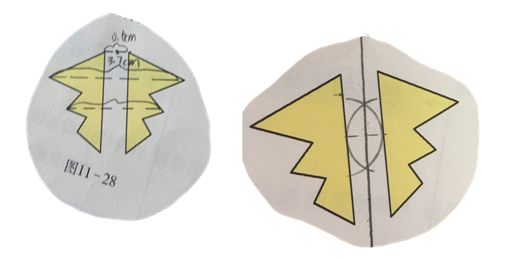# Shanghai Trip: Secondary take-aways

In November 2019, three teachers from the Cambridge Maths Hub visited a school in Shanghai to observe mathematics lessons and to talk to teachers. This is the first of a series of blogs that will explore some of the interesting things they encountered. It focuses on some ideas that cropped up in secondary lessons, as recorded by Ruth Colenso.

Idea 1: Using errors

The teacher photographed errors that were made throughout the topic and then used them at the end of the topic to do an overview – with students spotting the mistake and someone coming to the board to do it correctly.

Idea 2: Planning of a starter example/practice to feed into a later part of a lesson

In a lesson on circles, for the starter students had to find the circumference of two circles. The answers to these questions were written on the board (here is a reconstruction):Later in the lesson students had to find the radius of a circle when the circumference was given as 9.42m.

They could look at the earlier calculation and realise that it was the inverse, so the radius = 1.5

The teacher then looked at how you could efficiently work out the radius using an algebraic method:

The students could see that 6.28 came from 2π.

I like the idea that the teacher had planned the examples used in the starter so they would support work that would be carried out later in the lesson. Calculations being used in reverse is an idea that is common in Shanghai lessons.

Below is the board at the end of the lesson showing most of the work the class has covered, and the final question:Idea 3: Representations of mathematical ideas and when they need to change

To support children in understanding the ‘greater than’ and ‘less than’ symbols, I saw this representation.

1 is less than 4 can be shown using cubes:This works well until you want to consider negative numbers. At this point considering the position on the number line is more useful. As a way of understanding what the symbol ‘<’ means, the representation using cubes is helpful, though.

Idea 4: Relationship between fractions and ratio

Generalisation is a key feature of mathematics in Shanghai. The image shows the key vocabulary used in the first few ratio lessons, the basic properties of fractions and three algebraic representations (ratio, fraction and equivalent division) and how they are connected.This language and these representations are used from the start of year 7.

Idea 5: Forwards and Backwards – the power of the inverse

In Chinese lessons we saw expressions being explored both ways. For example, students know that

But in England we do not focus on the reverse: (which would help with understanding algebraic simplification).

In a reflection lesson students were reflecting shapes in a line:At the end of the lesson they had to do this in reverse and find the mirror line.

The first picture is a nearly finished version where the pupil reversed the method in the lesson. The second student chose to construct the perpendicular bisector.This shows connections between a method and its inverse and also a flexibility of approaches.

Read other Cambridge Maths Hub blogs here

Ruth Colenso Mark Dawes

Saffron Walden County High School Comberton Village College